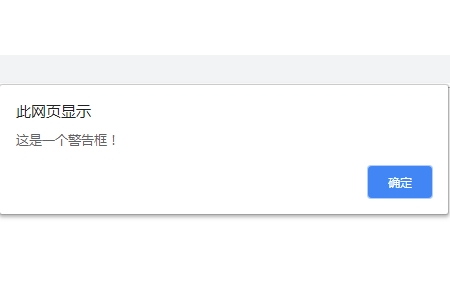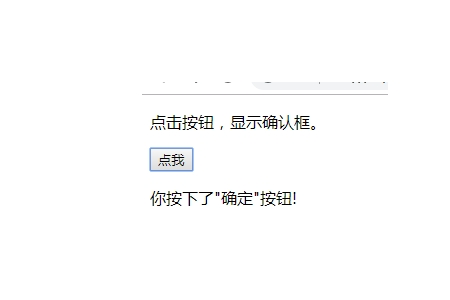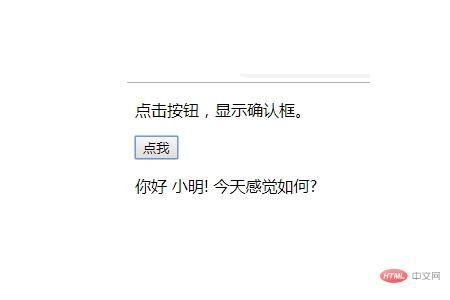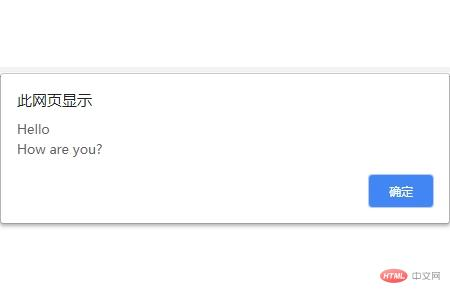JavaScript 弹窗

`window.alert("sometext");`

## 实例

```<script>
function myFunction()
{
}
</script>
<body>

<input type="button" onclick="myFunction()" value="显示警告框">````window.confirm("sometext");`

window.confirm() 方法可以不带上window对象，直接使用confirm()方法。

## 实例

```<script>
function myFunction(){
var x;
var r=confirm("按下按钮!");
if (r==true){
x="你按下了\"确定\"按钮!";
}
else{
x="你按下了\"取消\"按钮!";
}
document.getElementById("demo").innerHTML=x;
}
</script>````window.prompt("sometext","defaultvalue");`

window.prompt() 方法可以不带上window对象，直接使用prompt()方法。

## 实例

```<script>
function myFunction(){
var x;
var person=prompt("请输入你的名字","小明");
if (person!=null && person!=""){
x="你好 " + person + "! 今天感觉如何?";
document.getElementById("demo").innerHTML=x;
}
}
</script>```## 实例

```<script>
function myFunction(){